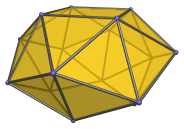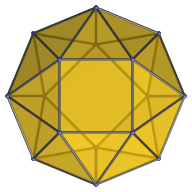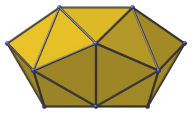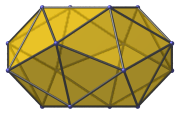# The Snub Square Antiprism

The snub square antiprism is the 85th Johnson solid (J85). Its surface consists of 24 equilateral triangles and 2 squares, for a total of 26 faces, 40 edges, and 16 vertices.The snub square antiprism is one of the special Johnson solids at the end of Norman Johnson's list that are not directly derived from the uniform polyhedra by cut-and-paste operations. It can be indirectly constructed from a square antiprism by separating it into two halves along a zigzag cut between its triangular faces, suitably deforming the two halves, and joining them together with a belt of 16 triangles.

An analogous process can be applied to the tetrahedron, treated as a digonal antiprism, to produce the snub disphenoid (J84). Applied to the octahedron, treated as a triangular antiprism, this process produces the icosahedron. It can also be applied to the pentagonal antiprism to produce a snub pentagonal antiprism; however, the result is no longer convex, and so is not among the Johnson solids.

## Projections

Here are some views of the snub square antiprism from various angles:

Projection DescriptionTop view.Side view.Oblique side view at 22.5° angle.

## Coordinates

The Cartesian coordinates of the snub square antiprism, centered on the origin with edge length 2, are:

• (±1, ±1, C)
• (±√2A, 0, B)
• (0, ±√2A, B)
• (±A, ±A, −B)
• (0, ±√2, −C)
• (±√2, 0, −C)

where A is the root of the following polynomial between 1.7 and 1.8:

A6 − 2A5 − 13A4 + 8A3 + 32A2 − 8A − 4 = 0

and:

 B = √(1 − (1 − 1/√2)A2) C = √(2(1 + √2A − A2)) + B

Their numerical values are approximately:

• A = 1.715731736910394
• B = 0.371214042564360
• C = 1.353737018062712

Last updated 18 Jun 2019.